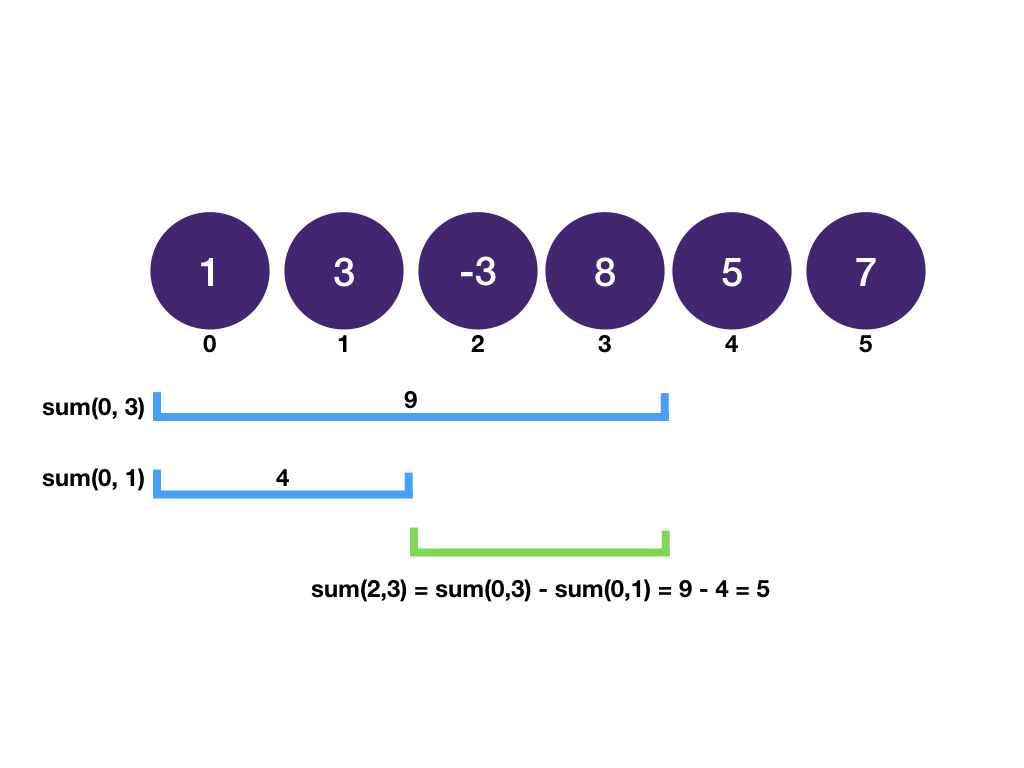# Subarray Sum

Given an array of integers and an integer `target`, find a subarray that sums to `target` and return the start and end indices of the subarray.

Input: `arr: 1 -20 -3 30 5 4` `target: 7`

Output: `1 4`

Explanation: `-20 - 3 + 30 = 7`. The indices for subarray `[-20,-3,30]` is `1` and `4` (right exclusive).

## Explanation

### Intuition

The brute force way is to find the sum of each subarray and compare it with the target. Let `N` be the number of elements in the array. There are `N` subarrays with size 1, `N-1` subarrays with size 2 .. and 1 subarray with size `N`. Time complexity is `O(N^2)`.

A key observation is that the the sum of a subarray `[i, j]` is equal to the sum of `[0, j]` minus the sum of `[0, i - 1]`.The sum of elements from index `0` to `i` is called the prefix sum. If we have the subarray sum for each index, we can find the sum of any subarray in constant time.

With the knowledge of the prefix sum under our belt, the problem boils down to Two Sum. We keep a dictionary of `prefix_sum: index` while going through the array calculating `prefix_sum`. If at any point, `prefix_sum - target` is in the dictionary, we have found our subarray.

Time Complexity: `O(n)`

## Solution

``````1from typing import List
2
3def subarray_sum(arr: List[int], target: int) -> List[int]:
4    # prefix_sum 0 happens when we have an empty array
5    prefix_sums = {0: 0}
6    cur_sum = 0
7    for i in range(len(arr)):
8        cur_sum += arr[i]
9        complement = cur_sum - target
10        if complement in prefix_sums:
11            return [prefix_sums[complement], i + 1]
12        prefix_sums[cur_sum] = i + 1
13
14if __name__ == '__main__':
15    arr = [int(x) for x in input().split()]
16    target = int(input())
17    res = subarray_sum(arr, target)
18    print(' '.join(map(str, res)))
19``````

Note that in the implementation, typically we use `prefix_sum[i]` to denote the sum of elements in `0...i - 1` (rightmost element `i` is not included in the sum). One good thing about this is `prefix_sum` then means sum of array up to but not including the first element, i.e. empty array. The definition of empty array sum is useful when there exists a subarray starting from 0 that sums up to the target. Without the definition of empty array sum we would miss it because its complement 0 does not exist in the dictionary.

Find the total number of subarrays that sums up to target.

## Explanation

Since the new problem does not ask for index but total number instead, we can change our hashmap to "sum k: number of prefix sums that sums up to k".

``````1from typing import Counter, List
2
3def subarray_sum_total(arr: List[int], target: int) -> int:
4    prefix_sums = Counter()
5    prefix_sums = 1 # since empty array's sum is 0
6    cur_sum = 0
7    count = 0
8    for i in range(len(arr)):
9        cur_sum += arr[i]
10        complement = cur_sum - target
11        if complement in prefix_sums:
12            count += prefix_sums[complement]
13        prefix_sums[cur_sum] += 1
14    return count
15
16if __name__ == '__main__':
17    arr = [int(x) for x in input().split()]
18    target = int(input())
19    res = subarray_sum_total(arr, target)
20    print(res)
21``````